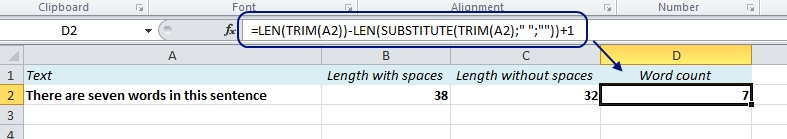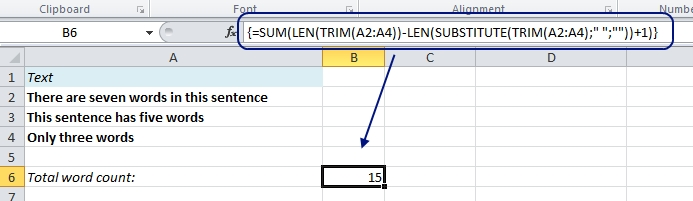# Use Excel to Count Words

In Word you can easily count the words simply by clicking “Word Count” from the Review ribbon. But what if you have your text in Excel? There is no built-in word count function in Excel, so we have to find another way.

In a previous post I showed how to count characters in a text string using the LEN function. We will use the same function, with a twist, to do a word count.

## Count words in one cell

First, let’s calculate the total length of the text in A2, including spaces:

=LEN(A2)

Then, let’s find out how long it would be without the spaces. We’ll use the SUBSTITUTE function to remove the spaces:

=LEN(SUBSTITUTE(A2,” “,””))

The difference between the two results – 6 – is the number of spaces between the words, so we have to add 1 to get the word count. If we combine the two formulas and add 1, we get this formula:

=LEN(A2)-LEN(SUBSTITUTE(A2,” “,””))+1

Sometimes there are extra spaces between the words or after the last word. You can use the TRIM function around the cell reference to get rid of them before you count the words:

=LEN(TRIM(A2))-LEN(SUBSTITUTE(TRIM(A2),” “,””))+1## Count words in multiple cells

If you want to do a word count on multiple cells, all you have to do is to change the cell reference (A2) to a range (A2:A4) and wrap the SUM function around it:

=SUM(LEN(TRIM(A2:A4))-LEN(SUBSTITUTE(TRIM(A2:A4),” “,””))+1)

This is an array formula, so you have to press Ctrl+Shift+Enter to make it work. The curly brackets only appear in the formula field to show that it’s an array formula – you don’t have to type it.More easy tricks:

Are you using a non-English version of Excel? Click here for translations of the 100 most common functions.